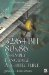# Function yABS(x) Absolute D A## Function y=ABS(x) 'Absolute' D = A

Floating-point FABS was discussed in Chapter 8, "Floating-Point Anyone?" Here we will investigate packed integer-based floating-point. There is sometimes found in C code a macro definition similar to:

` #define ABS(x) ((x) < 0 ? (x) : (x)) `

But it can be easily replaced in C without the conditional test:

` __inline int ABS(x) {     int y;     y = x >>  INT_MAX_BITS;     return (x ^ y)  y; } `

In the previous chapter on branching, you found the following example of the signed integer absolute number functiony=x, which uses a Jcc instruction.

` test eax,eax    ; Test if negative       jns  \$Abs       ; Jump if positive           neg  eax        ; Invert number, two's complement     \$Abs:                 ; eax is positive `

This can also be done without a Jcc instruction:

` mov   ecx,eax sar   ecx,INT_MAX_BITS   ; all one's if neg, else 0's xor   eax,ecx            ; At this point we're one's complement sub   eax,ecx            ; n(1)=n+1 = two's complement `

Voil , an ABS() function without any Jcc instructions. Just some sleight of hand using general-purpose instructions.

Let's look a little closer at how this works. Note that it will work on 8-, 16-, or 32-bit numbers in the same way. Below you will find an 8-bit negative number on the left and a positive 8-bit number on the right for a side-by-side comparison.

` mov cl,al  1  0001010b 8Ah (118)   mov dl,bl   1111011b 7Bh (123) sar cl,7  11111111  b FFh          sar dl,7  00000000  b 00h `

The SAR instruction shifts the MSB arithmetically into all the other bit positions , so a negative number becomes all FFs, and a positive number becomes all 00s. Now XOR those bits with their original XOR value. You learned in a previous chapter that 1 1=0 and 1 0=1.

` ; xor al,cl 01110101b 75h       xor bl,dl 01111011b 7Bh `

A negative value would actually flip the original value's bits with a one's complement, and a positive value would keep the value intact. We then subtract the new value with that mask, which effectively adds a +1 or +0, thus a two's complement is performed, since n(1)=n+1 = two's complement.

` ; cl=  11111111b  FFh         dl=  00000000  b 00h ; sub al,cl 01110110b 76h (118)   sub bl,dl 01111011b 7Bh (123) `

Pretty cool, huh! And no branching!32/64-Bit 80x86 Assembly Language Architecture
ISBN: 1598220020
EAN: 2147483647
Year: 2003
Pages: 191

Similar book on Amazon